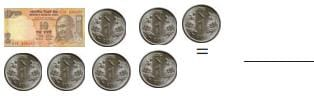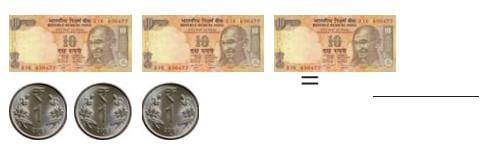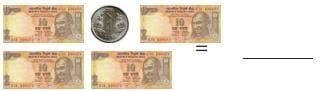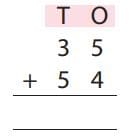Test: Tens And Ones - 1

# Test: Tens And Ones - 1

Test Description

## 10 Questions MCQ Test Mathematics for Class 2: NCERT | Test: Tens And Ones - 1

Test: Tens And Ones - 1 for Class 2 2023 is part of Mathematics for Class 2: NCERT preparation. The Test: Tens And Ones - 1 questions and answers have been prepared according to the Class 2 exam syllabus.The Test: Tens And Ones - 1 MCQs are made for Class 2 2023 Exam. Find important definitions, questions, notes, meanings, examples, exercises, MCQs and online tests for Test: Tens And Ones - 1 below.
Solutions of Test: Tens And Ones - 1 questions in English are available as part of our Mathematics for Class 2: NCERT for Class 2 & Test: Tens And Ones - 1 solutions in Hindi for Mathematics for Class 2: NCERT course. Download more important topics, notes, lectures and mock test series for Class 2 Exam by signing up for free. Attempt Test: Tens And Ones - 1 | 10 questions in 20 minutes | Mock test for Class 2 preparation | Free important questions MCQ to study Mathematics for Class 2: NCERT for Class 2 Exam | Download free PDF with solutions
 1 Crore+ students have signed up on EduRev. Have you?
Test: Tens And Ones - 1 - Question 1

### Write the total money for the following notes and coins.​​​​​​​Detailed Solution for Test: Tens And Ones - 1 - Question 1

Rs 10 notes = 1
= 10
Rupee 1 coins= 7
= 1 + 1 + 1 + 1 + 1 + 1 + 1

Total money = 10 + 1 + 1 + 1 + 1 + 1 + 1 + 1
= Rs 17

Test: Tens And Ones - 1 - Question 2

### Write the expanded form of 25.

Detailed Solution for Test: Tens And Ones - 1 - Question 2

25 = 20 + 5

Test: Tens And Ones - 1 - Question 3

### Write the short form of 90 + 4 =

Detailed Solution for Test: Tens And Ones - 1 - Question 3

90 + 4 = 94

Test: Tens And Ones - 1 - Question 4

Fill in the blank
40 = ___ + 0

Detailed Solution for Test: Tens And Ones - 1 - Question 4

40 = 40 + 0

Test: Tens And Ones - 1 - Question 5

Write the total money for the following notes and coins.Detailed Solution for Test: Tens And Ones - 1 - Question 5

Rs 10 notes = 3
= 10 + 10 + 10
Rupee 1 coins= 3
= 1 + 1 + 1

Total money = 10 + 10 + 10 + 1 + 1 + 1
= Rs 33

Test: Tens And Ones - 1 - Question 6

Write the expanded form of 99.

Detailed Solution for Test: Tens And Ones - 1 - Question 6

99 = 90 + 9

Test: Tens And Ones - 1 - Question 7

Write the short form of 80+7

Detailed Solution for Test: Tens And Ones - 1 - Question 7

80 + 7 = 87

Test: Tens And Ones - 1 - Question 8

Write the total money for the following notes and coins.Detailed Solution for Test: Tens And Ones - 1 - Question 8

Rs 10 notes = 4
= 10 + 10 + 10 + 10
Rupee 1 coins= 1
= 1

Total money = 10 + 10 + 10 + 10 + 1
= Rs 41

Test: Tens And Ones - 1 - Question 9

Fill in the blank
13 = ___ + 3

Detailed Solution for Test: Tens And Ones - 1 - Question 9

13 = 10 + 3

Test: Tens And Ones - 1 - Question 10Detailed Solution for Test: Tens And Ones - 1 - Question 10

35 + 54 = 89

## Mathematics for Class 2: NCERT

38 videos|62 docs|21 tests
 Use Code STAYHOME200 and get INR 200 additional OFF Use Coupon Code
Information about Test: Tens And Ones - 1 Page
In this test you can find the Exam questions for Test: Tens And Ones - 1 solved & explained in the simplest way possible. Besides giving Questions and answers for Test: Tens And Ones - 1, EduRev gives you an ample number of Online tests for practice

## Mathematics for Class 2: NCERT

38 videos|62 docs|21 tests